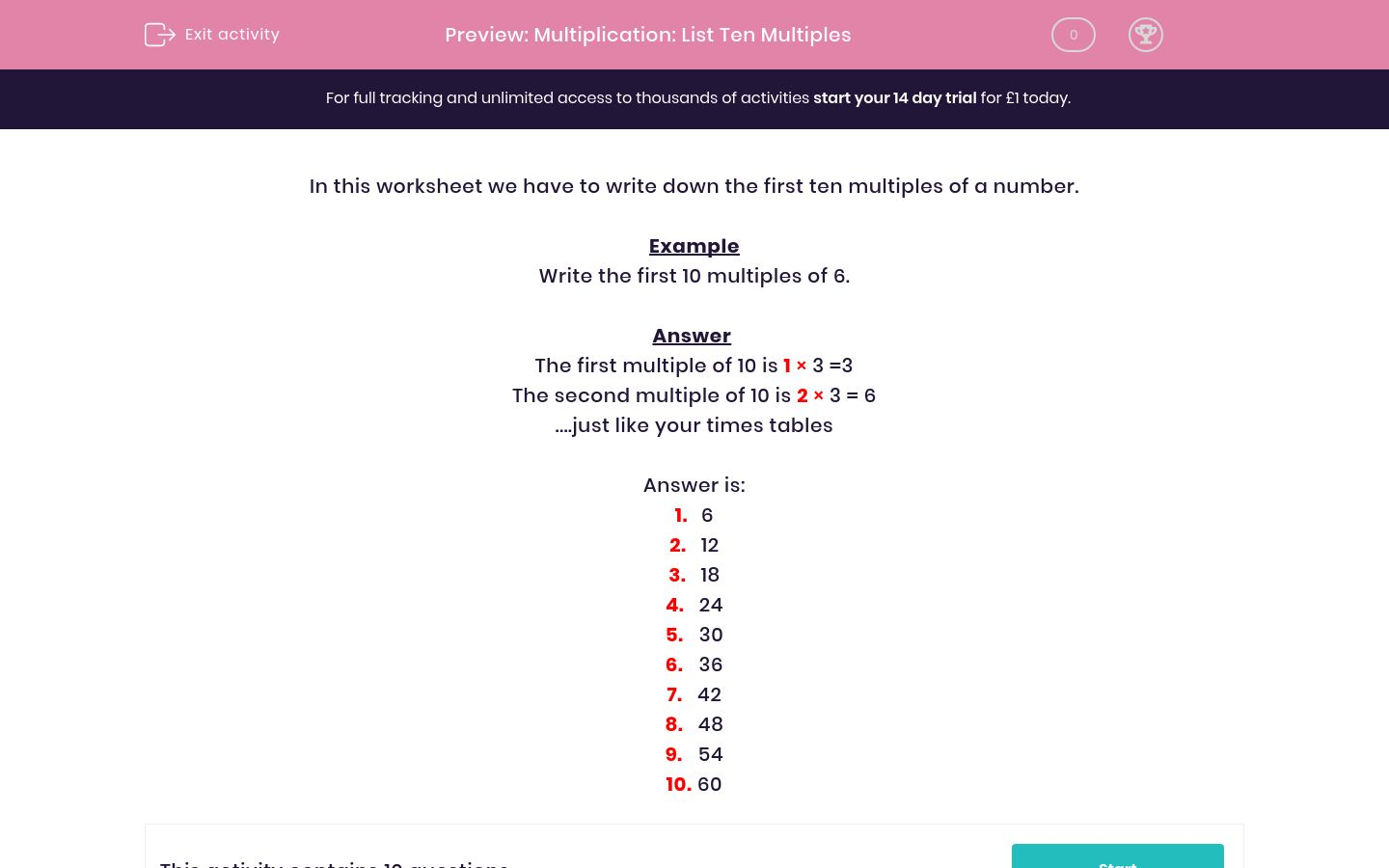# Multiplication: List Ten Multiples

In this worksheet, students write down the first ten multiples of the given numbers.Key stage:  KS 2

Curriculum topic:   Maths and Numerical Reasoning

Curriculum subtopic:   Factors and Multiples

Difficulty level:### QUESTION 1 of 10

In this worksheet we have to write down the first ten multiples of a number.

Example

Write the first 10 multiples of 6.

The first multiple of 10 is 1 × 3 =3

The second multiple of 10 is 2 × 3 = 6

1.   6

2.   12

3.   18

4.   24

5.   30

6.   36

7.   42

8.   48

9.   54

10. 60

Write the first 10 multiples of 5.

 Answers 1. 2. 3. 4. 5. 6. 7. 8. 9. 10.

Write the first 10 multiples of 4.

 Answers 1. 2. 3. 4. 5. 6. 7. 8. 9. 10.

Write the first 10 multiples of 2.

 Answers 1. 2. 3. 4. 5. 6. 7. 8. 9. 10.

Write the first 10 multiples of 10.

 Answers 1. 2. 3. 4. 5. 6. 7. 8. 9. 10.

Write the first 10 multiples of 8.

 Answers 1. 2. 3. 4. 5. 6. 7. 8. 9. 10.

Write the first 10 multiples of 9.

 Answers 1. 2. 3. 4. 5. 6. 7. 8. 9. 10.

Write the first 10 multiples of 3.

 Answers 1. 2. 3. 4. 5. 6. 7. 8. 9. 10.

Write the first 10 multiples of 6.

 Answers 1. 2. 3. 4. 5. 6. 7. 8. 9. 10.

Write the first 10 multiples of 7.

 Answers 1. 2. 3. 4. 5. 6. 7. 8. 9. 10.

Write the first 10 multiples of 9.

 Answers 1. 2. 3. 4. 5. 6. 7. 8. 9. 10.
• Question 1

Write the first 10 multiples of 5.

 Answers 1. 2. 3. 4. 5. 6. 7. 8. 9. 10.
• Question 2

Write the first 10 multiples of 4.

 Answers 1. 2. 3. 4. 5. 6. 7. 8. 9. 10.
• Question 3

Write the first 10 multiples of 2.

 Answers 1. 2. 3. 4. 5. 6. 7. 8. 9. 10.
• Question 4

Write the first 10 multiples of 10.

 Answers 1. 2. 3. 4. 5. 6. 7. 8. 9. 10.
• Question 5

Write the first 10 multiples of 8.

 Answers 1. 2. 3. 4. 5. 6. 7. 8. 9. 10.
• Question 6

Write the first 10 multiples of 9.

 Answers 1. 2. 3. 4. 5. 6. 7. 8. 9. 10.
• Question 7

Write the first 10 multiples of 3.

 Answers 1. 2. 3. 4. 5. 6. 7. 8. 9. 10.
• Question 8

Write the first 10 multiples of 6.

 Answers 1. 2. 3. 4. 5. 6. 7. 8. 9. 10.
• Question 9

Write the first 10 multiples of 7.

 Answers 1. 2. 3. 4. 5. 6. 7. 8. 9. 10.
• Question 10

Write the first 10 multiples of 9.

 Answers 1. 2. 3. 4. 5. 6. 7. 8. 9. 10.
---- OR ----

Sign up for a £1 trial so you can track and measure your child's progress on this activity.

### What is EdPlace?

We're your National Curriculum aligned online education content provider helping each child succeed in English, maths and science from year 1 to GCSE. With an EdPlace account you’ll be able to track and measure progress, helping each child achieve their best. We build confidence and attainment by personalising each child’s learning at a level that suits them.

Get started